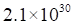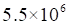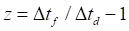Chapter 12, Problem 18P

Chapter
Section
Textbook Problem

. Sirius B has a mass and radius ofkg andm, respectively. Assuming Sirius B is perfectly spherical in shape, compute the ratio of theUsing this result, confirm that the redshift quoted in Section 12.2 for this star ifTo determine

The ratio of Δtf/Δtd.

Explanation

Given info:

m=2.1×1030kgr=5.5×106m

Formula used:

Δtf/Δtd=1[1( dsd)]12

ds=2GMc2,G=6.67×1011Nm2/kg2.

Calculation:

Substituting the given values, we get

ds=2×6.67×1011×2.1×1030(3× 108)2=3

Still sussing out bartleby?

Check out a sample textbook solution.

See a sample solution

The Solution to Your Study Problems

Bartleby provides explanations to thousands of textbook problems written by our experts, many with advanced degrees!

Get Started